# NEET (UG) – 2011 Pre Chemistry Previous Year

51. The total number of atomic orbitals in fourth energy level of an atom is

(1) 4                                (2) 8

(3) 16                              (4) 32

52.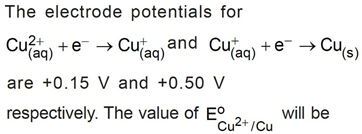(1) 0.150 V            (2) 0.500 V

(3) 0.325 V            (4) 0.650 V

Chemistry Notes

Physics Notes

Biology Notes

53. Mole fraction of the solute in a 1.00 molal aqueous solution is

(1) 1.7700                       (2) 0.1770

(3) 0.0177                       (4) 0.0344

54. By what factors does the average velocity of a gaseous molecule increase when the temperature (in Kelvin) is doubled?

(1) 1.4                             (2) 2.0

(3) 2.8                             (4) 4.0

54. By what factors does the average velocity of a gaseous molecule increase when the temperature (in Kelvin) is doubled?

(1) 1.4                             (2) 2.0

(3) 2.8                             (4) 4.0

Chemistry Notes

Physics Notes

Biology Notes

55. A buffer solution is prepared in which the concentration of NH3 is 0.30 M and the concentration of NH4 is 0.20 M. If the equilibrium constant, Kb for NH3 equals 1.8 × 10–5, what is the pH of this solution?

(1) 8.73                 (2) 9.08

(3) 9.43                 (4) 11.72

56. Two gases A and B having the same volume diffuse through a porous partition in 20 and 10 seconds respectively.  The molecular mass of A is 49 u. Molecular mass of B will be

(1) 25.00 u            (2) 50.00 u

(3) 12.25 u            (4) 6.50 u

Chemistry Notes

Physics Notes

Biology Notes

57. Which of the following is correct option for free expansion of an ideal gas under adiabatic condition?

(1) q = 0, ΔT < 0, w ≠ 0 (2) q = 0, ΔT ≠ 0, w = 0

(3) q ≠ 0, ΔT = 0, w = 0 (4) q = 0, ΔT = 0, w = 0

58. For the reaction N2(g) + O2(g) ⇌ 2NO(g) , the equilibrium constant is K1. The equilibrium constant is K2 for the reaction 2NO(g) + O2(g) ⇌ 2NO2(g) . What is K for the reaction NO (g) ½ N2 (g) ⇌ O2 (g)?

(a)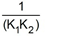(b)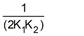(c)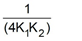(d)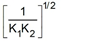Chemistry Notes

Physics Notes

Biology Notes

59. If x is amount of adsorbate and m is amount of adsorbent, which of the following relations is not related to adsorption process?

(1) x/m = p x T                          (2) x/m = f(p) at constant T

(3) x/m = f(T) at constant p       (4) p = f(T) at constant (x/m)

60. If the enthalpy change for the transition of liquid water to steam is 30 kJ mol–1 at 27°C, the entropy change for the process would be

(1) 100 J mol–1 K–1                    (2) 10 J mol–1 K–1

(3) 1.0 J mol–1 K–1                     (4) 0.1 J mol–1 K–1

61. The van’t Hoff factor i for a compound which undergoes dissociation in one solvent and association in other solvent is respectively

(1) Greater than one and greater than one

(2) Less than one and greater than one

(3) Less than one and less than one

(4) Greater than one and less than one

Chemistry Notes

Physics Notes

Biology Notes

62. Standard electrode potential for Sn4+ / Sn2+ couple is +0.15 V and that for the Cr3+ / Cr couple is –0.74 V. These two couples in their standard state are connected to make a cell. The cell potential will be

(1) +1.83 V           (2) +1.19 V

(3) +0.89 V           (4) +0.18 V

63. A gaseous mixture was prepared by taking equal mole of CO and N2. If the total pressure of the mixture was found 1 atmosphere, the partial pressure of the nitrogen (N2) in the mixture is

(1) 1 atm                (2) 0.5 atm

(3) 0.8 atm             (4) 0.9 atm

64. If the E0cell for a given reaction has a negative value, then which of the following gives the correct relationships for the values of ΔG° and Keq?

(a) ΔG° > 0; Keq < 1

(b) ΔG° > 0; Keq > 1

(c) ΔG° < 0; Keq > 1

(d) ΔG° < 0; Keq < 1

65. The freezing point depression constant for water is –1.86°Cm–1. If 5.00 g Na2SO4 is dissolved in 45.0 g H2O, the freezing point is changed by –3.82°C. Calculate the van’t Hoff factor for Na2SO4

(1) 0.381               (2) 2.05

(3) 2.63                 (4) 3.11

66. The energies E1 and E2 of two radiations are 25 eV and 50 eV respectively. The relation between their wavelengths i.e. λ1 and λ2 will be

(1) λ1 = ½ λ2                    (2) λ1 =  λ2

(3) λ1 = 2λ2                      (4) λ1 = 4λ2

67. Standard electrode potential of three metals X, Y and Z are –1.2 V, +0.5 V and –3.0 V respectively. The reducing power of these metals will be

(1) X > Y > Z                  (2) Y > Z > X

(3) Y > X > Z                  (4) Z > X > Y

Chemistry Notes

Physics Notes

Biology Notes

68. Which one of the following statements for the order of a reaction is incorrect?

(1) Order of reaction is always whole number

(2) Order can be determined only experimentally

(3) Order is not influenced by stoichiometric coefficient of the reactants

(4) Order of reaction is sum of power to the concentration terms of reactants to express the rate of reaction

69. Enthalpy change for the reaction, 4H(g) → 2H2(g) is –869.6 kJ. The dissociation energy of H – H bond is

(1) +217.4 kJ                   (2) –434.8 kJ

(3) –869.6 kJ                   (4) +434.8 kJ

70. If n = 6, the correct sequence of filling of electrons will be

(1) ns → np(n – 1)d → (n – 2)f

(2) ns → n(n –  2)f → (n – 1)d → np

(3) ns → (n – 1)d → (n – 2)f → np

(4) ns → (n – 2)f → np → (n – 1)d

71. Which of the following compounds has the lowest melting point?

(1) CaF2                           (2) CaCl2

(3) CaBr2                         (4) CaI2

72. Which of the following pairs of metals is purified by van Arkel method?

(1) Ni and Fe                   (2) Ga and In

(3) Zr and Ti                    (4) Ag and Au

Chemistry Notes

Physics Notes

Biology Notes

73. The correct order of increasing bond length of C – H, C – O, C – C and C = C is

(1) C – H < C – O < C – C < C = C

(2) C – H < C = C < C – O < C – C

(3) C – C < C = C < C – O < C – H

(4) C – O < C – H < C – C < C = C

74. Acidified K2Cr2O7 solution turns green when Na2SO3 is added to it. This is due to the formation of

(1) CrSO4                        (2) Cr2(SO4)3

(3) CrO42-                        (4) Cr2(SO3)3

75. For the four successive transition elements (Cr, Mn, Fe and Co), the stability of + 2 oxidation state will be there in which of the following order?

(1) Cr > Mn > Co > Fe

(2) Mn > Fe > Cr > Co

(3) Fe > Mn > Co > Cr

(4) Co > Mn > Fe > Cr